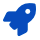Science - Physics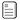Course Preface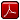Printable Version (pdf)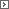Course Introduction

Core Standards of the Course

Science Benchmark
The motion of an object can be described by measurements of its position at different times. Velocity is a measure of the rate of change of position of an object. Acceleration is a measure of the rate of change of velocity of an object. This change in velocity may be a change in speed and/or direction. Motion is defined relative to the frame of reference from which it is observed. An object’s state of motion will remain constant unless unbalanced forces act upon the object. This is Newton’s first law of motion.

Standard 1
Students will understand how to measure, calculate, and describe the motion of an object in terms of position, time, velocity, and acceleration.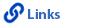Objective 1
Describe the motion of an object in terms of position, time, and velocity.1. Calculate the average velocity of a moving object using data obtained from measurements of position of the object at two or more times.
2. Distinguish between distance and displacement.
3. Distinguish between speed and velocity.
4. Determine and compare the average and instantaneous velocity of an object from data showing its position at given times.
5. Collect, graph, and interpret data for position vs. time to describe the motion of an object and compare this motion to the motion of another object.

Objective 2
Analyze the motion of an object in terms of velocity, time, and acceleration.1. Determine the average acceleration of an object from data showing velocity at given times.
2. Describe the velocity of an object when its acceleration is zero.
3. Collect, graph, and interpret data for velocity vs. time to describe the motion of an object.
4. Describe the acceleration of an object moving in a circular path at constant speed (i.e., constant speed, but changing direction).
5. Analyze the velocity and acceleration of an object over time.

Objective 3
Relate the motion of objects to a frame of reference.1. Compare the motion of an object relative to two frames of reference.
2. Predict the motion of an object relative to a different frame of reference (e.g., an object dropped from a moving vehicle observed from the vehicle and by a person standing on the sidewalk).
3. Describe how selecting a specific frame of reference can simplify the description of the motion of an object.

Objective 4
Use Newton's first law to explain the motion of an object.1. Describe the motion of a moving object on which balanced forces are acting.
2. Describe the motion of a stationary object on which balanced forces are acting.
3. Describe the balanced forces acting on a moving object commonly encountered (e.g., forces acting on an automobile moving at constant velocity, forces that maintain a body in an upright position while walking).

Science Benchmark
Objects in the universe interact with one another by way of forces. Changes in the motion of an object are proportional to the sum of the forces, and inversely proportional to the mass. If one object exerts a force on a second object, the second object always exerts an equal and opposite force on the first object. Whenever a force is applied to an object there is an equal and opposite reaction force.

Any two objects in the universe with mass exert equal and opposite gravitational forces on one another. The electromagnetic force is manifested as an electric force, a magnetic force, or a combination. Any two objects in the universe with a net electric charge exert equal and opposite electric forces on one another. While gravitational forces are always attractive, electromagnetic forces can be either attractive or repulsive.

Friction, tension, compression, spring, gravitational, and normal forces are all common observable forces. The net force on an object is the vector sum of all the forces acting upon the object.

Standard 2
Students will understand the relation between force, mass, and acceleration.Objective 1
Analyze forces acting on an object.1. Observe and describe forces encountered in everyday life (e.g., braking of an automobile - friction, falling rain drops - gravity, directional compass - magnetic, bathroom scale - elastic or spring).
2. Use vector diagrams to represent the forces acting on an object.
3. Measure the forces on an object using appropriate tools.
4. Calculate the net force acting on an object.

Objective 2
Using Newton’s second law, relate the force, mass, and acceleration of an object.1. Determine the relationship between the net force on an object and the object’s acceleration.
2. Relate the effect of an object’s mass to its acceleration when an unbalanced force is applied.
3. Determine the relationship between force, mass, and acceleration from experimental data and compare the results to Newton’s second law.
4. Predict the combined effect of multiple forces (e.g., friction, gravity, and normal forces) on an object’s motion.

Objective 3
Explain that forces act in pairs as described by Newton’s third law.1. Identify pairs of forces (e.g., action-reaction, equal and opposite) acting between two objects (e.g., two electric charges, a book and the table it rests upon, a person and a rope being pulled).
2. Determine the magnitude and direction of the acting force when magnitude and direction of the reacting force is known.
3. Provide examples of practical applications of Newton’s third law (e.g., forces on a retaining wall, rockets, walking).
4. Relate the historical development of Newton’s laws of motion to our current understanding of the nature of science (e.g., based upon previous knowledge, empirical evidence, replicable observations, development of scientific law).

Standard 3
Students will understand the factors determining the strength of gravitational and electric forces.Objective 1
Relate the strength of the gravitational force to the distance between two objects and the mass of the objects (i.e., Newton’s law of universal gravitation).1. Investigate how mass affects the gravitational force (e.g., spring scale, balance, or other method of finding a relationship between mass and the gravitational force).
2. Distinguish between mass and weight.
3. Describe how distance between objects affects the gravitational force (e.g., effect of gravitational forces of the moon and sun on objects on Earth).
4. Explain how evidence and inference are used to describe fundamental forces in nature, such as the gravitational force.
5. Research the importance of gravitational forces in the space program.

Objective 2
Describe the factors that affect the electric force (i.e., Coulomb’s law).1. Relate the types of charge to their effect on electric force (i.e., like charges repel, unlike charges attract).
2. Describe how the amount of charge affects the electric force.
3. Investigate the relationship of distance between charged objects and the strength of the electric force.
4. Research and report on electric forces in everyday applications found in both nature and technology (e.g., lightning, living organisms, batteries, copy machine, electrostatic precipitators).

Science Benchmark
The total energy of the universe is constant; however, the total amount of energy available for useful transformation is almost always decreasing. Energy can be converted from one form to another and move from one system to another. Transformation of energy usually produces heat that spreads to cooler places by radiation, convection, or conduction. Energy can be classified as potential or kinetic energy. Potential energy is stored energy and includes chemical, gravitational, electrostatic, elastic, and nuclear. Kinetic energy is the energy of motion.

Moving electric charges produce magnetic forces and moving magnets produce electric forces. The interplay of electric and magnetic forces is the basis for electric motors, generators, and many other modern technologies, including the production of electromagnetic waves. Modern electric generators produce electricity by converting mechanical energy into electrical energy.

Sound and light transfer energy from one location to another as waves. Characteristics of waves include wavelength, amplitude, and frequency. Waves can combine with one another, bend around corners, reflect off surfaces, be absorbed by materials they enter, and change direction when entering a new material. All these effects vary with wavelength. Observable waves include mechanical and electromagnetic waves. Mechanical waves transport energy through a medium. Electromagnetic radiation is differentiated by wavelength or frequency, and includes radio waves, microwaves, infrared, visible light, ultraviolet radiation, x-rays, and gamma rays. These wavelengths vary from radio waves (the longest) to gamma rays (the shortest). In empty space all electromagnetic waves move at the same speed, the “speed of light.”

Standard 4
Students will understand transfer and conservation of energy.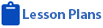Objective 1
Determine kinetic and potential energy in a system.1. Identify various types of potential energy (i.e., gravitational, elastic, chemical, electrostatic, nuclear).
2. Calculate the kinetic energy of an object given the velocity and mass of the object.
3. Describe the types of energy contributing to the total energy of a given system.

Objective 2
Describe conservation of energy in terms of systems.1. Describe a closed system in terms of its total energy.
2. Relate the transformations between kinetic and potential energy in a system (e.g., moving magnet induces electricity in a coil of wire, roller coaster, internal combustion engine).
3. Gather data and calculate the gravitational potential energy and the kinetic energy of an object (e.g., pendulum, water flowing downhill, ball dropped from a height) and relate this to the conservation of energy of a system.
4. Evaluate social, economic, and environmental issues related to the production and transmission of electrical energy.

Objective 3
Describe common energy transformations and the effect on availability of energy.1. Describe the loss of useful energy in energy transformations.
2. Investigate the transfer of heat energy by conduction, convection, and radiation.
3. Describe the transformation of mechanical energy into electrical energy and the transmission of electrical energy.
4. Research and report on the transformation of energy in electrical generation plants (e.g., chemical to heat to electricity, nuclear to heat to mechanical to electrical, gravitational to kinetic to mechanical to electrical), and include energy losses during each transformation.

Standard 5
Students will understand the properties and applications of waves.Objective 1
Demonstrate an understanding of mechanical waves in terms of general wave properties.1. Differentiate between period, frequency, wavelength, and amplitude of waves.
2. Investigate and compare reflection, refraction, and diffraction of waves.
3. Provide examples of waves commonly observed in nature and/or used in technological applications.
4. Identify the relationship between the speed, wavelength, and frequency of a wave.
5. Explain the observed change in frequency of a mechanical wave coming from a moving object as it approaches and moves away (i.e., Doppler effect).
6. Explain the transfer of energy through a medium by mechanical waves.

Objective 2
Describe the nature of electromagnetic radiation and visible light.1. Describe the relationship of energy to wavelength or frequency for electromagnetic radiation.
2. Distinguish between the different parts of the electromagnetic spectrum (e.g., radio waves and x-rays or visible light and microwaves).
3. Explain that the different parts of the electromagnetic spectrum all travel through empty space and at the same speed.
4. Explain the observed change in frequency of an electromagnetic wave coming from a moving object as it approaches and moves away (i.e., Doppler effect, red/blue shift).
5. Provide examples of the use of electromagnetic radiation in everyday life (e.g., communications, lasers, microwaves, cellular phones, satellite dishes, visible light).http://www.uen.org - in partnership with Utah State Board of Education (USBE) and Utah System of Higher Education (USHE).  Send questions or comments to USBE Specialist - Richard Scott and see the Science - Secondary website. For general questions about Utah's Core Standards contact the Director - Jennifer Throndsen .

These materials have been produced by and for the teachers of the State of Utah. Copies of these materials may be freely reproduced for teacher and classroom use. When distributing these materials, credit should be given to Utah State Board of Education. These materials may not be published, in whole or part, or in any other format, without the written permission of the Utah State Board of Education, 250 East 500 South, PO Box 144200, Salt Lake City, Utah 84114-4200.